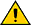MathScript RT Module Functions

LabVIEW 2012 MathScript RT模块帮助

»查看产品信息下载帮助（仅限Windows）

Requires: MathScript RT Module

Use the LabVIEW MathScript functions to perform mathematical or signal processing calculations and analysis using a text-based environment. You can use LabVIEW MathScript to write functions and scripts for use in the LabVIEW MathScript Window or MathScript Node. The following is a list of all classes of functions and commands that LabVIEW MathScript supports.

(LabVIEW 64-bit) LabVIEW MathScript is not supported in LabVIEW (64-bit).Caution  (Real-Time Module) National Instruments does not guarantee and is not responsible for the jitter characteristics of code you run on an RT target. Depending on the functions and data types you use, non-deterministic function calls might be required at run time, which can increase jitter and lead to unbounded execution time in your real-time application. However, you can achieve time-bounded execution using MathScript RT Module functions on RT targets if you follow certain guidelines. To ensure that the application meets timing requirements, National Instruments recommends that you review your code and benchmark the jitter in your application before you deploy the application to the field. You are solely responsible for testing the jitter characteristics of your application.

The LabVIEW Control Design and Simulation Module installs additional MathScript RT Module functions.

The LabVIEW Digital Filter Design Toolkit installs additional MathScript RT Module functions.

Class Description
approximation Approximation and interpolation
audio Sound functions
basic Basic mathematical functions
bitwise Bit-oriented functions
boolean Boolean functions
commands Commands
comparison Relational operators
communications Manipulate signals
constants Constants
daq Data acquisition
dsp Digital signal processing
filter design Filter design
filter implementation Filter implementation
geometry Combinatorial geometry
ignored Ignored functions
integration Integration
linalgebra Linear algebra
linear systems Linear systems
matrix Special matrices
matrixops Matrix operators
membership Membership
modeling and prediction Modeling and prediction
ode ODE solvers
optimization Optimization and minimization
pde Partial differential equation solvers
plots Plot functions
polynomials Polynomial functions
programming Programming constructs
resampling Resampling functions
rt RT debugging and timing functions
sets Set operators
spectral analysis Spectral analysis
statistics Statistics
string String functions
struct Related data
support Support functions
time Date and time functions
timing Timing functions
transforms Transforms
trigonometric Trigonometric functions
vector Vector analysis
waveform generation Waveform generation
windows Window generation
zerofinder Zeros of a function

﻿